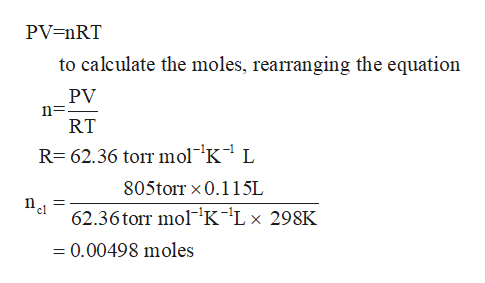# Chlorine can be prepared in the laboratory by the reaction of manganese dioxide with hydrochloric acid, HCl(aq)HCl(aq), as described by the chemical equation MnO2(s)+4HCl(aq)⟶MnCl2(aq)+2H2O(l)+Cl2(g)MnO2(s)+4HCl(aq)⟶MnCl2(aq)+2H2O(l)+Cl2(g) How much MnO2(s)MnO2(s) should be added to excess HCl(aq)HCl(aq) to obtain 115 mL Cl2(g)115 mL Cl2(g) at 25 °C and 805 Torr805 Torr?

Question
5049 views

Chlorine can be prepared in the laboratory by the reaction of manganese dioxide with hydrochloric acid, HCl(aq)HCl(aq), as described by the chemical equation

MnO2(s)+4HCl(aq)⟶MnCl2(aq)+2H2O(l)+Cl2(g)MnO2(s)+4HCl(aq)⟶MnCl2(aq)+2H2O(l)+Cl2(g)

How much MnO2(s)MnO2(s) should be added to excess HCl(aq)HCl(aq) to obtain 115 mL Cl2(g)115 mL Cl2(g) at 25 °C and 805 Torr805 Torr?

check_circle

Step 1

Given values

Volume of chlorine to be obtained = 115ml

Temperature = 25°C

= (25+ 273.15)K = 298.15K

Pressure = 805 torr

Step 2

Volume of Cl2= 115ml

Since 1ml = 0.001L

Therefore, volume of Cl2= 0.115L

Step 3

According to ideal ...help_outlineImage TranscriptionclosePV=nRT to calculate the moles, rearranging the equation PV RT R 62.36 torr mol'K-1 L 805torr x 0.115L Bel 62.36torr mol'K'Lx 298K 0.00498 moles fullscreen

### Want to see the full answer?

See Solution

#### Want to see this answer and more?

Solutions are written by subject experts who are available 24/7. Questions are typically answered within 1 hour.*

See Solution
*Response times may vary by subject and question.
Tagged in

### Quantitative analysis